Equilibria - Phase, Chemical & Ionic

# Equilibria - Phase, Chemical & Ionic

Test Description

## 20 Questions MCQ Test GATE Chemistry Mock Test Series | Equilibria - Phase, Chemical & Ionic

Equilibria - Phase, Chemical & Ionic for Chemistry 2022 is part of GATE Chemistry Mock Test Series preparation. The Equilibria - Phase, Chemical & Ionic questions and answers have been prepared according to the Chemistry exam syllabus.The Equilibria - Phase, Chemical & Ionic MCQs are made for Chemistry 2022 Exam. Find important definitions, questions, notes, meanings, examples, exercises, MCQs and online tests for Equilibria - Phase, Chemical & Ionic below.
Solutions of Equilibria - Phase, Chemical & Ionic questions in English are available as part of our GATE Chemistry Mock Test Series for Chemistry & Equilibria - Phase, Chemical & Ionic solutions in Hindi for GATE Chemistry Mock Test Series course. Download more important topics, notes, lectures and mock test series for Chemistry Exam by signing up for free. Attempt Equilibria - Phase, Chemical & Ionic | 20 questions in 60 minutes | Mock test for Chemistry preparation | Free important questions MCQ to study GATE Chemistry Mock Test Series for Chemistry Exam | Download free PDF with solutions
 1 Crore+ students have signed up on EduRev. Have you?
Equilibria - Phase, Chemical & Ionic - Question 1

### If K < Q. where, K = equilibrium constant. Q = reaction quotient The reaction move in which direction to achieve equilibrium

Detailed Solution for Equilibria - Phase, Chemical & Ionic - Question 1

Application of equilibrium constant
K > Q ⇒ forward direction
K < Q ⇒ backward direction
K = Q ⇒ at equilibrium

Equilibria - Phase, Chemical & Ionic - Question 2

### For the reaction,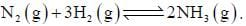On increasing pressure equilibrium shift to

Detailed Solution for Equilibria - Phase, Chemical & Ionic - Question 2

Le-Chatelier’s principle. On increasing pressure equilibrium shift in the directions where pressure decrease and so in this case it moves in forward direction.

Equilibria - Phase, Chemical & Ionic - Question 3

### The effect of which variable is ignored for the study of two-component system

Detailed Solution for Equilibria - Phase, Chemical & Ionic - Question 3

Reduced phase rule,
F = C - P + 1
Pressure is ignored as only condensed phases studied for two-components system

Equilibria - Phase, Chemical & Ionic - Question 4

A sample of propane (C3H8) placed in a closed vessel together with an amount of O2, that is 3-times the amount needed to completely oxidize the propane to CO2 and H2O at constant temperature. Calculate the mole of fraction of each component in the resulting mixture after oxidation assuming that the H2O is present as a gas?

Detailed Solution for Equilibria - Phase, Chemical & Ionic - Question 4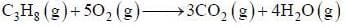Let initially'm' mole of propane present when reaction is complete then
nO2 =15 m mol
When reaction is complete then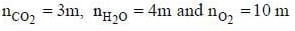∴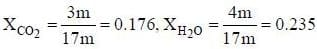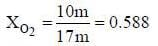Equilibria - Phase, Chemical & Ionic - Question 5

For the below diagram, which statement is correct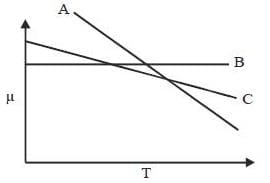Detailed Solution for Equilibria - Phase, Chemical & Ionic - Question 5

dG = VdP - SdT + μdN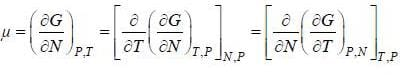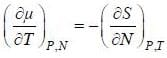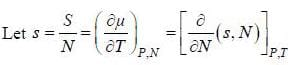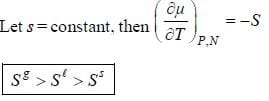Equilibria - Phase, Chemical & Ionic - Question 6

A solution is 0.1M in CI- and 0.001M in CrO2-4. If solid AgNO3, is gradually added to this solution, the one which precipitate first is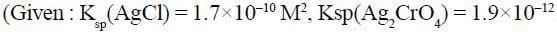Detailed Solution for Equilibria - Phase, Chemical & Ionic - Question 6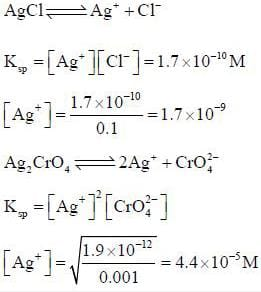Since amount of Ag+ needed to precipitate AgCl is less therefore, it will precipitate out first.

*Answer can only contain numeric values
Equilibria - Phase, Chemical & Ionic - Question 7

At 39.9°C. a solution of ethanol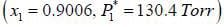and iso-octane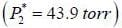foms a vappour phase with Y1 = 0.6667 at a total pressure of 185.9 torr. The activity coefficient of ethanol is __________ (Hint: X1 = mole fraction in solution, Y1 = mole fraction vapour phase) (Round off to two decimal places).

Detailed Solution for Equilibria - Phase, Chemical & Ionic - Question 7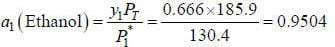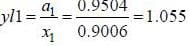Equilibria - Phase, Chemical & Ionic - Question 8

Which of the following graph are correct?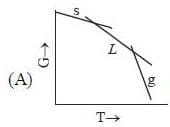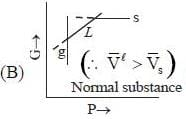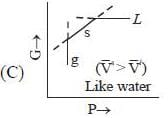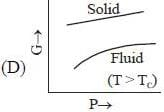Detailed Solution for Equilibria - Phase, Chemical & Ionic - Question 8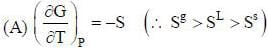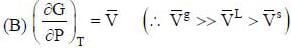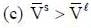( a temperature lower than the triple point temperature)
(d) Gibb's energies at a temperature greater than critical temperature this case G(P) varies smoothly with P. There is no discontinuity in the slope in tliis case because only a single fluid phase is involved.

Equilibria - Phase, Chemical & Ionic - Question 9

Phase-diagram that shown below represents the congruent melting point. Which of the following statements are not correct?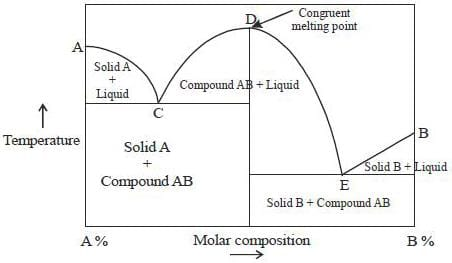(A) There will be three freezing point curves
(B) Congruent melting point always lies above the melting point of pure components
(C) Congruent melting point diagram always contains equimolar amounts of A and B
(D) There are two eutectic point (in above figure)

Detailed Solution for Equilibria - Phase, Chemical & Ionic - Question 9

(A) Yes, Ac. BE and C'DE are three freezing point curve.
(B) Melting point of the compound formed is not always lie above it may be above, below and in-betweeen them elting point of the pure components.
(C) It is not necessary that the congruent melting point phase diagram always contains equimolar of both component
(D) C and E are 2-Eutectic point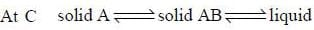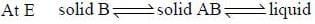(Therefore. Eutectinc point means easy melt's)

*Answer can only contain numeric values
Equilibria - Phase, Chemical & Ionic - Question 10

In an ideal solution of A and B 3.50 mol are in the liquid phase and 4.75 mol are in the gaseous phase. The overall composition of the system is ZA = 0.300 and XA = 0.250. The mole fraction in vapour phase of YA is ____________(round off to two decimal places).

Detailed Solution for Equilibria - Phase, Chemical & Ionic - Question 10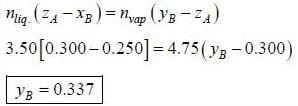Equilibria - Phase, Chemical & Ionic - Question 11

For the equilibrium,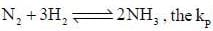is expressed as

Detailed Solution for Equilibria - Phase, Chemical & Ionic - Question 11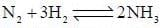Total number of moles = 1 + 3 + 2 = 6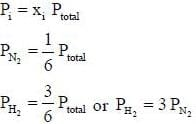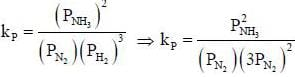⇒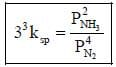Equilibria - Phase, Chemical & Ionic - Question 12

The degree of freedom for a two component system, at congruent melting point is

Detailed Solution for Equilibria - Phase, Chemical & Ionic - Question 12

Degree of freedom at congruent melting point is zero.

Equilibria - Phase, Chemical & Ionic - Question 13

For an indicator, the value of pH is 2 when half of the indicator is present in unionised form. The percentage of indicator in unionised form in a solution of 4.0x10-3 M hydrogen ion concentration is

Detailed Solution for Equilibria - Phase, Chemical & Ionic - Question 13

For indicators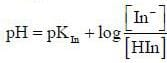When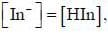pH = 2
⇒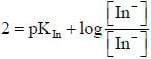Or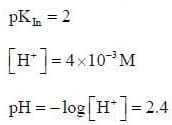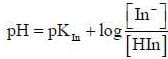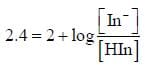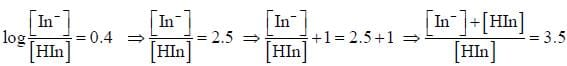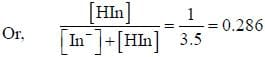Therefore, 28.6% is present in unionised form.

Equilibria - Phase, Chemical & Ionic - Question 14

For a system A (s) + B(g)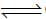C(l) + D(s). The number of phases are

Equilibria - Phase, Chemical & Ionic - Question 15

A solution is made-up of 184.2g of ethanol and 108.1 g of H2O, if the volume of the solution is 333.4 cm3 and the partial molar volume of H2O,  is 17 cm3. What is the partial molar volume of ethanol under these conditions?

Detailed Solution for Equilibria - Phase, Chemical & Ionic - Question 15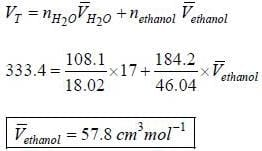Equilibria - Phase, Chemical & Ionic - Question 16

The number of component for the reaction,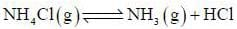in vacuum is

Detailed Solution for Equilibria - Phase, Chemical & Ionic - Question 16

C = N - r where, N = number of chemical constituted
N = 3
r = 2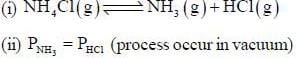Hence, C = 3-2 = 1

Equilibria - Phase, Chemical & Ionic - Question 17

The pH of 10-7 HCI solution is

Detailed Solution for Equilibria - Phase, Chemical & Ionic - Question 17

H2O ↔↔ H+ + OH-
These H+ from water contribute to total hydrogen ion concentration. Hence, the hydrogen ion concentration increases from 10-7 M and pH decreases from 7 as
[H+] = 2 × 10-7 M
pH = - log​[H+] = 6.69

*Answer can only contain numeric values
Equilibria - Phase, Chemical & Ionic - Question 18

The pH of a solution made by adding 0.001 mole of NaOH to 100cmof a solution which is 0.50 M in CH3COOH and 0.50 M in CH3COONa is _________________ (pkCH3cooH = 4.744) (Round off to two decimal places).

Detailed Solution for Equilibria - Phase, Chemical & Ionic - Question 18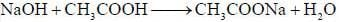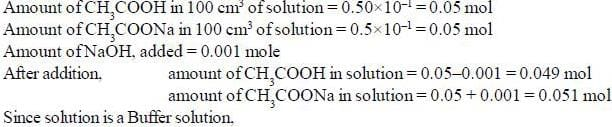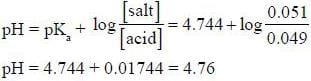*Answer can only contain numeric values
Equilibria - Phase, Chemical & Ionic - Question 19

The vapour pressure of solid and liquid chlorine is given by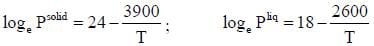where Psolid and p liquid are vapour pressure (in Torr) of solid and liquid chlorine near the triple point absolute temperature. The ratio of slope of solid-gas curve to the slope of liquid-gas curve at the trip the P-T diagram is ______(Round off to one decimal places).

Detailed Solution for Equilibria - Phase, Chemical & Ionic - Question 19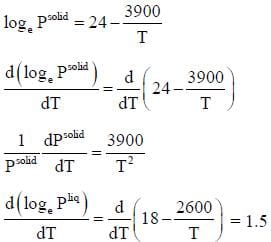*Answer can only contain numeric values
Equilibria - Phase, Chemical & Ionic - Question 20

At a certain temperature, k= 0.11 for the reaction,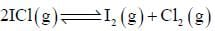The equilibrium concentration of IC1 if 0.75 mole of Iand 0.75 mol of CIare initially mixed in 2L flask is ___mol/L. (Round off to two decimal places).

Detailed Solution for Equilibria - Phase, Chemical & Ionic - Question 20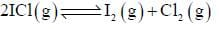kc = 0.11
For the reaction,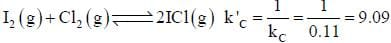Now,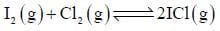Initial concntration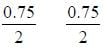0
Equilibrium concentration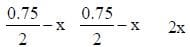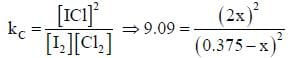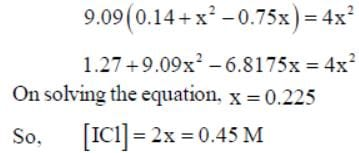## GATE Chemistry Mock Test Series

1 docs|34 tests
 Use Code STAYHOME200 and get INR 200 additional OFF Use Coupon Code
Information about Equilibria - Phase, Chemical & Ionic Page
In this test you can find the Exam questions for Equilibria - Phase, Chemical & Ionic solved & explained in the simplest way possible. Besides giving Questions and answers for Equilibria - Phase, Chemical & Ionic, EduRev gives you an ample number of Online tests for practice

1 docs|34 tests